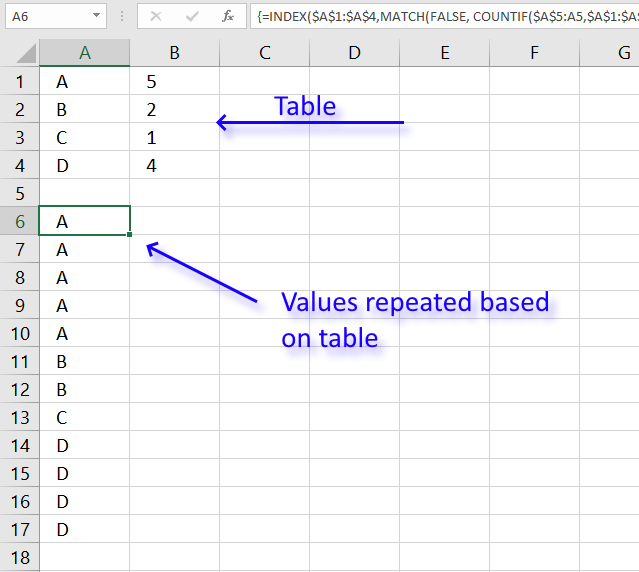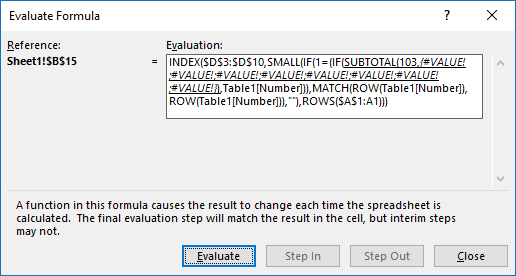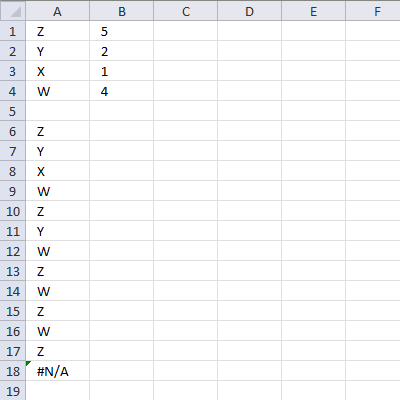Author: Oscar Cronquist Article last updated on January 16, 2023This article explains how to repeat specific values based on a table, the table contains the items to be repeated and how many times they will be repeated.

The array formula in cell A6 utilizes the table and repeats the values until the condition is met.

There is also a section below that explains how to repeat values in sequence based on corresponding numbers.

I failed to find the right article in your blog and therefore I want to ask you in newest post. So I have a table similar to this:
A 5
B 2
C 1
D 4
Is it possible with a formula to generate a list like this:
A
A
A
A
A
B
B
C
D
D
D
D

Array formula in cell A6:

=INDEX(\$A\$1:\$A\$4, MATCH(FALSE, COUNTIF(\$A\$5:A5, \$A\$1:\$A\$4)=\$B\$1:\$B\$4, 0))

### How to create an array formula

1. Double press with left mouse button on cell A6 so the prompt appears.
2. Copy and paste the array formula above to the cell.
3. Press and hold CTRL + SHIFT simultaneously.
4. Press Enter once.
5. Release all keys.

You can check using the formula bar that you did above steps right, Excel tells you if a cell contains an array formula by surrounding the formula with a beginning and ending curly brackets, like this: {=array_formula}.

Don't enter these characters yourself they show up automatically if you did the above steps correctly.

### Explaining array formula in cell A6I recommend that you use the built-in "Evaluate Formula" feature in Excel to better understand and troubleshoot formulas, it is a great tool that allows you to see each calculation step.

Go to tab "Formulas" on the ribbon, then press with left mouse button on "Evaluate Formula" button. Press with left mouse button on "Evaluate" button to move to next calculation step, press with left mouse button on "Close" button to dismiss the dialog box.

#### Step 1 - Count previous values

The COUNTIF function counts values in a cell range based on a condition, however, in this case, I use a growing cell reference that expands when you copy the cell and paste to cells below.

This will make the COUNTIF function count based on multiple conditions, this technique is what makes you required to enter the formula as an array formula.

This way the function keeps track of previous values above the current cell so the correct number of values is returned based on the table.

COUNTIF(\$A\$5:A5,\$A\$1:\$A\$4)

COUNTIF(rangecriteria)

The first argument is \$A\$5:A5 which is a cell reference, however, it contains two parts. The first part is an absolute cell reference pointing to A5, you can tell that it is absolute by the \$ characters.

It means that the cell reference is locked to cell A5, both the column and row number is, locked, and does not change when you copy the cell to cells below. There are exceptions to this like inserting new rows or columns.

The second part is a relative cell reference and this changes when you copy the cell and paste to cells below which is great because the cell reference expands and takes multiple cells into account.

For example, in cell A6 the cell reference is \$A\$5:A5, however, in cell A7 the cell reference changes to \$A\$5:A6 and this makes the formula aware of previous returned values above the current cell.

COUNTIF(\$A\$5:A5,\$A\$1:\$A\$4)

becomes

COUNTIF("",{"A";"B";"C";"D"})

and returns {0;0;0;0}

In cell A6 there are no previous values above, only cell A5 which is empty.

The COUNTIF function returns an array containing the same number of values as the table and each value in the array represents how many times the values in the table have been displayed.

An array uses commas and semicolons to separate values, commas are between columns and semicolons are between rows.

#### Step 2 - Compare array values to list

This step checks if the values in the array is equal to the numbers in the table, this works because the values in the array correspond to the numbers in the table.

The equal sign compares the returned values from the COUNTIF function with the values in cell range B1:B4 and returns TRUE or FALSE. Note that the cell reference to B1:B4 is absolute. We don't want it to change when the cell is copied to cells below.

COUNTIF(\$A\$5:A5,\$A\$1:\$A\$4)=\$B\$1:\$B\$4

becomes

{0;0;0;0}={5;2;1;4}

and returns {FALSE;FALSE;FALSE;FALSE}

Not a single value meets the criteria in the table in cell A6, that is why all values in the array are FALSE.

#### Step 3 - Match first FALSE value in array

The MATCH function returns the position of a given value in a cell range or array.

MATCH(lookup_valuelookup_array[match_type])

The first argument is FALSE, we want to know the position of the first value that has not been repeated the number of times specified in the table.

The second argument is the array we created in step 2 and the third argument is 0 (zero) which means we want an exact match.

MATCH(FALSE, COUNTIF(\$A\$5:A5,\$A\$1:\$A\$4)=\$B\$1:\$B\$4,0)

becomes

MATCH(FALSE, {FALSE;FALSE;FALSE;FALSE},0)

and returns 1.

#### Step 4 - Return a value of the cell at the intersection of a particular row and column

The INDEX function returns a value from a cell range or array based on a row and column number. The column number is optional if the cell range is only a single column.

INDEX(\$A\$1:\$A\$4, MATCH(FALSE, COUNTIF(\$A\$5:A5, \$A\$1:\$A\$4)=\$B\$1:\$B\$4, 0))

becomes

=INDEX(\$A\$1:\$A\$4, 1)

and returns A in cell A6.

### Get the Excel filerepeat-values.xlsx

### Repeat values in a predetermined seriesThis example demonstrates a formula that repeats values in a given sequence and also how many times each value is to be repeated.

Hi Oscar,
Great Job.. Is this possible to repeat the range according to criteria in loop.. like below.Z 5
Y 2
X 1
W 4then Z 5 Times, Y 2 Times.... but in series
Z
Y
X
W
Z
Y
W
Z
W
Z
W
ZRegards,
Deb

Array formula in cell A6:

=INDEX(\$A\$1:\$A\$4, MATCH(MIN(IF(COUNTIF(\$A\$5:A5, \$A\$1:\$A\$4)=\$B\$1:\$B\$4, "", COUNTIF(\$A\$5:A5, \$A\$1:\$A\$4))), IF(COUNTIF(\$A\$5:A5, \$A\$1:\$A\$4)=\$B\$1:\$B\$4, "", COUNTIF(\$A\$5:A5, \$A\$1:\$A\$4)), 0))

### Explaining formula in cell A6

#### Step 1 - Count previous values

COUNTIF(\$A\$5:A5, \$A\$1:\$A\$4)=\$B\$1:\$B\$4

becomes

{0;0;0;0}=\$B\$1:\$B\$4

becomes

{0;0;0;0}={5;2;1;4}

and returns

{FALSE;FALSE;FALSE;FALSE}.

#### Step 2 - Replace values to be repeated with their count

IF(COUNTIF(\$A\$5:A5, \$A\$1:\$A\$4)=\$B\$1:\$B\$4, "", COUNTIF(\$A\$5:A5, \$A\$1:\$A\$4))

becomes

IF({FALSE; FALSE; FALSE; FALSE}, "", {0;0;0;0})

and returns

{0;0;0;0}.

#### Step 3 - Find smallest count number

MIN(IF(COUNTIF(\$A\$5:A5, \$A\$1:\$A\$4)=\$B\$1:\$B\$4, "", COUNTIF(\$A\$5:A5, \$A\$1:\$A\$4)))

becomes

MIN({0;0;0;0})

and returns 0 (zero).

#### Step 4 - Find relative position

MATCH(MIN(IF(COUNTIF(\$A\$5:A5, \$A\$1:\$A\$4)=\$B\$1:\$B\$4, "", COUNTIF(\$A\$5:A5, \$A\$1:\$A\$4))), IF(COUNTIF(\$A\$5:A5, \$A\$1:\$A\$4)=\$B\$1:\$B\$4, "", COUNTIF(\$A\$5:A5, \$A\$1:\$A\$4)), 0)

becomes

MATCH(0, IF(COUNTIF(\$A\$5:A5, \$A\$1:\$A\$4)=\$B\$1:\$B\$4, "", COUNTIF(\$A\$5:A5, \$A\$1:\$A\$4)), 0)

becomes

MATCH(0, {0;0;0;0}, 0)

and returns 1.

#### Step 5 - Return value based on position

INDEX(\$A\$1:\$A\$4, MATCH(MIN(IF(COUNTIF(\$A\$5:A5, \$A\$1:\$A\$4)=\$B\$1:\$B\$4, "", COUNTIF(\$A\$5:A5, \$A\$1:\$A\$4))), IF(COUNTIF(\$A\$5:A5, \$A\$1:\$A\$4)=\$B\$1:\$B\$4, "", COUNTIF(\$A\$5:A5, \$A\$1:\$A\$4)), 0))

becomes

INDEX(\$A\$1:\$A\$4, 1)

and returns "Z" in cell A6.

### Get the Excel filerepeat-values-v2.xlsx